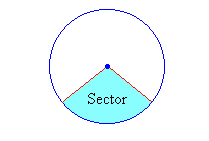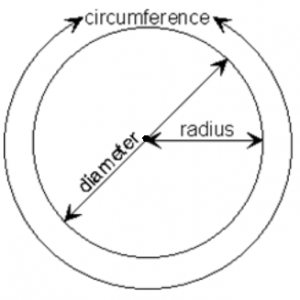## How to Calculate and Solve for the Perimeter or Circumference, Diameter, Radius and Angle of a Sector | The Calculator EncyclopediaThe image above is a sector.

To compute the Perimeter or Circumference a sector, two essential parameters is needed and this parameters are the radius of the sector (r) and the angle of the sector (θ). You can also use the diameter of the sector (d).

The formula for calculating the Perimeter or Circumference of a sector:

P = 2r + θ / 360(2πr)

Where;

P = Perimeter or Circumference
r = Radius of the sector
θ = Angle of the Sector

Let’s solve an example:
Find the perimeter or circumference of a sector when the radius of the sector is 14 cm and the angle of the sector is 60°

This implies that;

r = Radius of the sector = 14 cm
θ = Angle of the sector = 60°

P = 2r + θ / 360(2πr)
P = 2 x 14 + 60 / 360(2 x 3.142 x 14)
P = 28 + 0.1667 (87.97)
P = 28 + 14.66
P = 42.66

Therefore, the perimeter or circumference of the sector is 42.66 cm.

Calculating the Perimeter or Circumference of a Sector using Diameter and Angle of the sector.

P = d + θ / 360(πd)

θ = Angle of the sector
d = Diameter of the sector

Let’s solve an example;
Find the perimeter or circumference of a sector when the diameter of the sector is 20 cm and the angle of the sector is 80°.

This implies that;

d = Diameter of the sector = 20 cm
θ = Angle of the sector = 80°

## How to Calculate and Solve for the Perimeter or Circumference, Radius and Diameter of a Circle | The Calculator EncyclopediaThe image above is a circle.

To compute the perimeter or circumference of a circle, one essential parameter is needed and this parameter is the radius of a circle (r). You can also use the diameter of a circle to compute the area of a circle (d).

The formula for calculating the perimeter or circumference of a circle is:

P = 2πr

Where:

P = Perimeter or Circumference of a circle
r = Radius of a circle

Let’s solve an example:
Find the perimeter or circumference of a circle where the radius of a circle is 8 cm.

This implies that;
r = Radius of a circle = 8 cm

P = 2πr
P = 2 x 3.142 x 8
P = 50.265

Therefore, the perimeter or circumference of a circle is 50.265 cm.

Calculating the Area of a Circle using the Diameter of a Circle.

The formula is P = πd

Where:

P = Perimeter or Circumference of a circle
d = Diameter of a circle

Let’s solve an example:
Find the perimeter or circumference of a circle where the diameter of a circle is 10 cm.

This implies that;
d = Diameter of a circle = 10 cm

P = πd
P = 3.142 x 10
P = 31.42

Therefore, the perimeter or circumference of a circle with diameter is 31.42 cm.

How to Calculate Radius of a Circle when Perimeter or Circumference of the Circle is Given

r = P /

where;

r = Radius of a circle
P = Perimeter or Circumference of a circle

Let’s solve an example:
Find the radius of a circle where the perimeter or circumference of the circle is 16 cm.

This implies that;
P = Perimeter or Circumference of the circle = 16 cm

r = P /
r = 16 / 6.284
r = 2.55

Therefore, the radius of the circle is 2.55 cm.

How to Calculate Diameter of a Circle when Perimeter or Circumference of the Circle is Given

d = P / π

where;

d = Diameter of a circle
P = Perimeter or Circumference of a circle

Let’s solve an example;
Find the diameter of a circle where the perimeter or circumference of the circle is 20 cm

This implies that;
P = Perimeter or Circumference of the circle = 20 cm

d = P / π
d = 20 / π
d = 6.365

Therefore, the diameter of the circle is 6.365 cm.